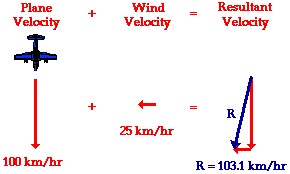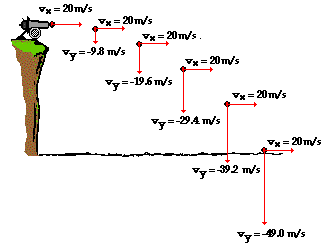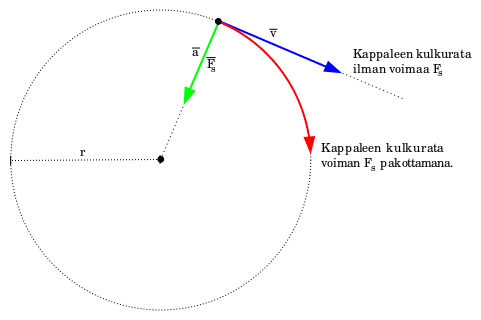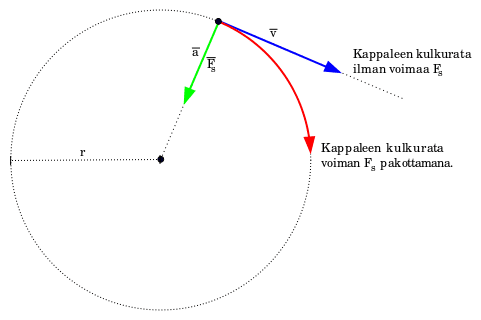# Why doesn't centripetal force change the magnitude of speed

sameeralord
Hello guys,

It is hard for me to explain my question so I'll use a diagramConsider plane velocity - As centripetal force
Wind velocity- As the velocity of object at the start
R- As the resultant velocity

So in the centripetal force objects this resultant velocity seems constant? How can this be when I add the vectors and use maths as in this question?

Thank you so muchMentor
In order for a force to change an object's direction of motion, but not its speed, that force must be always perpendicular to the object's velocity. That's not the case in your example. As soon as the wind acts, it changes the plane's velocity so that it is no longer perpendicular to the force.

sameeralord
In order for a force to change an object's direction of motion, but not its speed, that force must be always perpendicular to the object's velocity. That's not the case in your example. As soon as the wind acts, it changes the plane's velocity so that it is no longer perpendicular to the force.

Thank you so much for taking your time to help me Doc AlHowever I'm still confused, I'm rereading your response to grasp this. Have I labelled the variables wrong. Could you please tell me how you would label the variables? Thank youIn this example if the question says what is the speed of the ball at second circle, I have to add horizonatl and vertical components so how is the speed of the ball constant?

Last edited:
Mentor
Thank you so much for taking your time to help me Doc AlHowever I'm still confused, I'm rereading your response to grasp this. Have I labelled the variables wrong. Could you please tell me how you would label the variables? Thank youThe issue has nothing to do with labels. For the wind to change the plane's direction but not its speed, the wind would have to shift so that it's always hitting the plane sideways to its direction of motion. That's not the case here. This is not an example of centripetal force.

Here's an example that illustrates what you're looking for, I think. Attach a ball on the end of a string and twirl it in a horizontal circle. The horizontal component of the string tension provides the centripetal force. That force is always perpendicular to the ball's velocity, which is tangent to the circle. As the ball moves around in a circle, the force changes direction to stay perpendicular to the ball's velocity vector.

MentorIn this example if the question says what is the speed of the ball at second circle, I have to add horizonatl and vertical components so how is the speed of the ball constant?
The speed of the ball is not constant. It's falling!

Only the horizontal component of the velocity is constant, since gravity only acts vertically.

Staff Emeritus
Thank you so much for taking your time to help me Doc AlHowever I'm still confused, I'm rereading your response to grasp this. Have I labelled the variables wrong. Could you please tell me how you would label the variables? Thank youIn this example if the question says what is the speed of the ball at second circle, I have to add horizonatl and vertical components so how is the speed of the ball constant?

The gravitational force is normal to the velocity vector only at the instant the cannonball comes out of the cannon. This is similar to the situation Doc Al described in post #2. Paraphrasing his response, "As soon as gravity acts, it changes the cannonball's velocity so that it is no longer perpendicular to the gravitational force."

sameeralord
Thanks Doc Al and DH for your helpIf the force is acting perpendicular to the velocity all the time how can it change the direction? Why doesn't centripetal force change the direction of the ball velocity so it is not perpendicular to the centripetal force?

Is this projectile motion completely irrevelant to centripetal force. Can I understand the answer to my question from the diagram I posted?

Sorry I know I have made a mess of this.

Mentor
If the force is acting perpendicular to the velocity all the time how can it change the direction?
It pushes it sideways. In the example with the ball on a string, the ball is constantly changing direction.
Why doesn't centripetal force change the direction of the ball velocity so it is not perpendicular to the centripetal force?
The ball's velocity and the centripetal force change direction together at just the right rate so that they stay perpendicular.

Is this projectile motion completely irrevelant to centripetal force.
Yes. (Unless the projectile is moving so fast that it goes into orbit!)
Can I understand the answer to my question from the diagram I posted?
Which question? The problem with the cannon ball speed? Sure.

sameeralord
It pushes it sideways. In the example with the ball on a string, the ball is constantly changing direction.

Thanks again Doc AlNow this is my misunderstanding. If you look at the second circle in my cannon diagram this is what I'm thinking. The ball velocity is 20m/s and the speed from centripetal force is 9.8 m/s, and the resultant velocity is the vector sum of that? Could you please help using this example.Thank youMentor
The ball velocity is 20m/s and the speed from centripetal force is 9.8 m/s, and the resultant velocity is the vector sum of that?
Yes, but there's no 'centripetal force' in this problem, just gravity acting down. Vx = 20 m/s and at the end of 1 second Vy = -9.8 m/s. What's the resultant velocity?

sameeralord
Yes, but there's no 'centripetal force' in this problem, just gravity acting down. Vx = 20 m/s and at the end of 1 second Vy = -9.8 m/s. What's the resultant velocity?

Ok but the resultant velocity is not going to be 20m/s so how is centripetal force changing direction without magnitude? Thanks a lot for helping so farsameeralord
Yes, but there's no 'centripetal force' in this problem, just gravity acting down. Vx = 20 m/s and at the end of 1 second Vy = -9.8 m/s. What's the resultant velocity?

Ok but the resultant velocity is not going to be 20m/s so how is centripetal force changing direction without magnitude? Thanks a lot for helping so farI'm considering the blue line as 20 m/s and the green line as 9.8 m/s.

Mentor
Ok but the resultant velocity is not going to be 20m/s so how is centripetal force changing direction without magnitude?
Listen carefully: This is a projectile motion problem. Centripetal force has nothing to do with it! This is not an example of circular motion and centripetal force.

Mentor
Ok but the resultant velocity is not going to be 20m/s so how is centripetal force changing direction without magnitude? Thanks a lot for helping so farI'm considering the blue line as 20 m/s and the green line as 9.8 m/s.
This diagram is not relevant to this problem. There's no circular motion or centripetal force here. It's a projectile motion problem!

sameeralord
Listen carefully: This is a projectile motion problem. Centripetal force has nothing to do with it! This is not an example of circular motion and centripetal force.

I think I just edited my post while you were replying. Could you please help me with the new diagram? Thank youMentor
See my comments above regarding the new diagram.